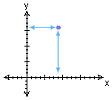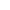Go to contentCoordinates (x,y) give the position of a point on a graph. The x coordinate tells you how far across and the y coordinate tells you how far up.

The coordinates (2,3) indicate a point 2 units to the right of the origin and 3 units up.
The coordinates (-4,-1) indicate a point 4 units to the left of the origin and 1 unit down.

Some people remember this as "along the corridor and up the stairs" or "x is a cross, wise up!"You can get a better display of the maths by downloading special TeX fonts from jsMath. In the meantime, we will do the best we can with the fonts you have, but it may not be pretty and some equations may not be rendered correctly.

## Glossary

### coordinate

One of the measurements of distance of a point from the axes of the graph.

### graph

A diagram showing a relationship between two variables.
The diagram shows a vertical y axis and a horizontal x axis.

### origin

The point from where all measurements of coordinates are made; usually the point where the two axes of a graph cross.

### union

The union of two sets A and B is the set containing all the elements of A and B.

Full Glossary List

## This question appears in the following syllabi:

SyllabusModuleSectionTopicExam Year
AQA GCSE (9-1) Foundation (UK)A: GraphsA8: Understanding CoordinatesCoordinates in All Four Quadrants-
CBSE IX (India)Coordinate GeometryCoordinate GeometryCoordinates-
CIE IGCSE (9-1) Maths (0626 UK)5 Co-ordinate GeometryC5.1 Cartesian Co-ordinatesCoordinates in All Four Quadrants-
Edexcel GCSE (9-1) Foundation (UK)A: GraphsA8: Understanding CoordinatesCoordinates in All Four Quadrants-
GCSE Foundation (UK)AlgebraCoordinatesFinding coordinates-
OCR GCSE (9-1) Foundation (UK)7: Graphs of Equations and Functions7.01a: x- and y-CoordinatesCoordinates in All Four Quadrants-
Universal (all site questions)CCoordinatesFinding coordinates-